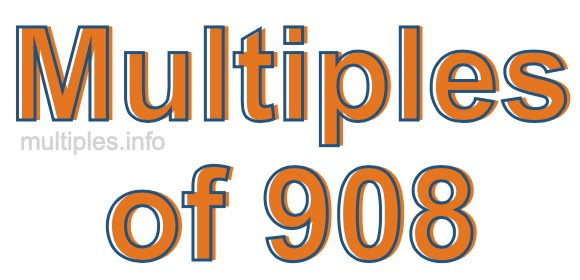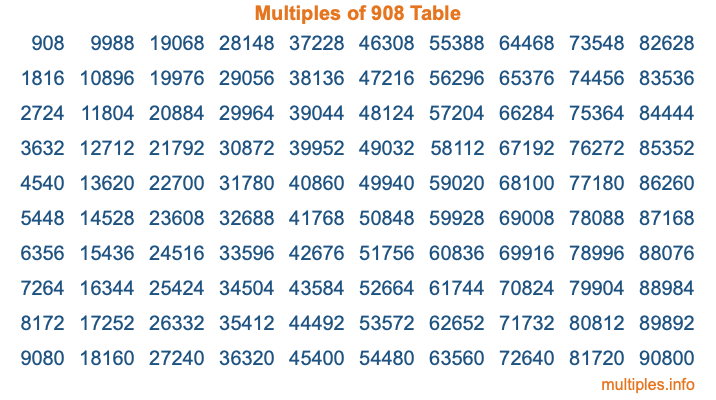Multiples of 908Welcome to the Multiples of 908 page. Here we will first teach you everything you will ever need to know about the multiples of 908, and then give you a study guide summary of everything we taught you to make sure you remember it all. Use this page to look up facts and learn information about the multiples of 908. This page will make you a multiples of nine hundred eight expert!

Definition of Multiples of 908
Multiples of 908 are all the numbers that when divided by 908 equal an integer. Each of the multiples of 908 are called a multiple. A multiple of 908 is created by multiplying 908 by an integer.

Therefore, to create a list of multiples of 908, you start with 1 multiplied by 908, then 2 multiplied by 908, then 3 multiplied by 908, and so on for as long as you want. Thus, the list of the first five multiples of 908 is 908, 1816, 2724, 3632, and 4540. To see a larger list of multiples of 908, see the printable image of Multiples of 908 further down on this page. We also have a category where you can choose any nth multiple of 908.

Multiples of 908 Checker
The Multiples of 908 Checker below checks to see if any number of your choice is a multiple of 908. In other words, it checks to see if there is any number (integer) that when multiplied by 908 will equal your number. To do that, we divide your number by 908. If the the quotient is an integer, then your number is a multiple of 908.

Is  a multiple of 908?

Least Common Multiple of 908 and ...
A Least Common Multiple (LCM) is the lowest multiple that two or more numbers have in common. This is also called the smallest common multiple or lowest common multiple and is useful to know when you are adding our subtracting fractions. Enter one or more numbers below (908 is already entered) to find the LCM.

Check out our LCM Calculator if you need more details about the Least Common Multiple or if you need the LCM for different numbers for adding and subtraction fractions.

nth Multiple of 908
As we stated above, 908 is the first multiple of 908, 1816 is the second multiple of 908, 2724 is the third multiple of 908, and so on. Enter a number below to find the nth multiple of 908.

th multiple of 908

Multiples of 908 vs Factors of 908
908 is a multiple of 908 and a factor of 908, but that is where the similarities end. All postive multiples of 908 are 908 or greater than 908. All positive factors of 908 are 908 or less than 908.

Below is the beginning list of multiples of 908 and the factors of 908 so you can compare:

Multiples of 908: 908, 1816, 2724, 3632, 4540, etc.

Factors of 908: 1, 2, 4, 227, 454, 908

As you can see, the multiples of 908 are all the numbers that you can divide by 908 to get a whole number. The factors of 908, on the other hand, are all the whole numbers that you can multiply by another whole number to get 908.

It's also interesting to note that if a number (x) is a factor of 908, then 908 will also be a multiple of that number (x).

Multiples of 908 vs Divisors of 908
The divisors of 908 are all the integers that 908 can be divided by evenly. Below is a list of the divisors of 908.

Divisors of 908: 1, 2, 4, 227, 454, 908

The interesting thing to note here is that if you take any multiple of 908 and divide it by a divisor of 908, you will see that the quotient is an integer.

Multiples of 908 Table
Below is an image of the first 100 multiples of 908 in a table. The table is in chronological order, column by column. The first column has the first ten multiples of 908, the second column has the next ten multiples of 908, and so on.The Multiples of 908 Table is also referred to as the 908 Times Table or Times Table of 908. You are welcome to print out our table for your studies.

Negative Multiples of 908
Although not often discussed or needed in math, it is worth mentioning that you can make a list of negative multiples of 908 by multiplying 908 by -1, then by -2, then by -3, and so on, to get the following list of negative multiples of 908:

-908, -1816, -2724, -3632, -4540, etc.

Multiples of 908 Summary
Below is a summary of important Multiples of 908 facts that we have discussed on this page. To retain the knowledge on this page, we recommend that you read through the summary and explain to yourself or a study partner why they hold true.

There are an infinite number of multiples of 908.

A multiple of 908 divided by 908 will equal a whole number.

908 divided by a factor of 908 equals a divisor of 908.

The nth multiple of 908 is n times 908.

The largest factor of 908 is equal to the first positive multiple of 908.

908 is a multiple of every factor of 908.

908 is a multiple of 908.

A multiple of 908 divided by a divisor of 908 equals an integer.

908 divided by a divisor of 908 equals a factor of 908.

Any integer times 908 will equal a multiple of 908.

Multiples of a Number
Here you can get the multiples of another number, all with the same attention to detail as we did for multiples of 908 on this page.

Multiples of
Multiples of 909
Did you find our page about multiples of nine hundred eight educational? Do you want more knowledge? Check out the multiples of the next number on our list!